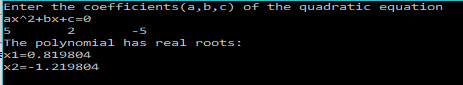# C Program to find the roots of a Quadratic EquationIn this post I show you how to write a C program that finds the roots(both real and complex) of a quadratic equation.

### Theory:

A quadratic equation looks like this:$ax^2+bx+c=0$

We will therefore, ask the user to enter the coefficients($a,b,c$).

The discriminant is given as,$d=b^2-4ac$

If$d=0$ then the equation has real and equal roots, if$d>0$ then the equation has real and unequal roots, and if$d<0$ then the equation has complex roots.

So, in our program, we will first perform a check to find out if the roots are going to be real or complex by using the above conditions.

Then accordingly we will, use the following formulas to find the root:

Real Roots($d>=0$):$root1=\frac{-b+\sqrt{b^2-4ac}}{2a}$$root2=\frac{-b-\sqrt{b^2-4ac}}{2a}$

Complex Roots($d<0$):$root1=\frac{-b}{2a} + i(\frac{\sqrt{b^2-4ac}}{2a})$$root2=\frac{-b}{2a} - i(\frac{\sqrt{b^2-4ac}}{2a})$

That’s all the information you need to be able to write the program.

### Program:

/**************************************
2017 (c) Manas Sharma - https://bragitoff.com
*************************************/
#include<stdio.h>
#include<math.h>
main()
{
double a,b,c,root1,root2,d,imag;
printf("Enter the coefficients(a,b,c) of the quadratic equation\nax^2+bx+c=0\n");
scanf("%lf%lf%lf",&a,&b,&c);
//printf("%f,%f,%f",a,b,c);
d=b*b-4*a*c;
if(d>=0){
/****REAL ROOTS CALCULATION****/
root1=(-b+sqrt(d))/(2*a);
root2=(-b-sqrt(d))/(2*a);
printf("The polynomial has real roots:\nx1=%f\nx2=%f\n",root1,root2);
}
else{
/****COMPLEX ROOTS CALCULATION****/
root1=-b/(2*a);
imag=sqrt(fabs(d))/(2*a);
printf("The polynomial has complex  roots:\nx1=%f+%fi\nx2=%f-%fi\n",root1,imag,root1,imag);
}
}


### OUTPUT:[wpedon id="7041" align="center"]